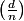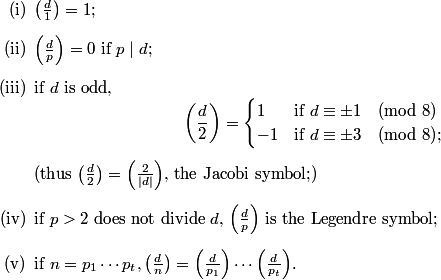### Evaluating the Kronecker symbolHere d is an integer and n is a positive integer.

We use the definition of the Kronecker symbol contained in Henri Cohen's book A Course in Computational Algebraic Number Theory. As usual, p denotes a prime.This symbol is used in Dirichlet's class number formula, where d is a fundamental discriminant.

E. Landau, in common with some other authors, in his definition on page 51 of Elementare Zahlentheorie, adds the restriction that d ≡ 0 or 1 (mod 4) and also assumes that d is not a perfect square, with the latter assumption only being used in Satz 99,5), which states that= -1 for some n.

Also see R.G. Ayoub, An Introduction to the Analytic Theory of Numbers, 1963, page 290.

This is a BCMATH version of a BC program.

Enter an integer d:
Enter a positive integer n: